# 长期记忆神经图灵机Long-Term Memory Neural Turing Machines

• 全文下载: PDF(714KB)    PP.49-58   DOI: 10.12677/CSA.2018.81008
• 下载量: 670  浏览量: 2,173

The readable and writable external memory module can improve the ability of the neural network which is based on factual memory and memory-based reasoning. Neural Turing Machines use attention mechanism to design a reading and writing mechanism for the memory module. It also realized sorting, copying and other algorithms by using a recurrent neural network as a controller. To shorten the training time and speed up convergence in a wider range of applications (such as natural language processing), we design a kind of read-write mechanism based on the global memory which is applied in Turing machines. This kind of read-write mechanism does convolution operations to extract the global memory features, whose training speed is 6 times better than Neural Turing Machines; the convergence rate and reasoning results in the bAbi dataset are also better.

1. 引言

2. 相关工作

2.1. 神经图灵机

2.2. 可微分神经计算机

DNC将神经图灵机的推理能力提高到了更高的层次，同时复杂的结构设计以及对输入信息的处理上使DNC在对待普通任务时同样会受到训练过长、收敛速度慢的问题。

2.3. 记忆神经网络

3. 模型结构

3.1. 基础结构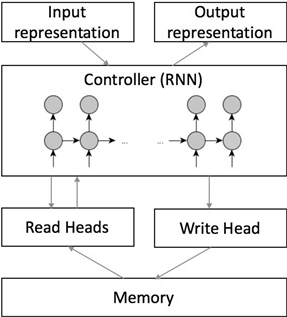Figure 1. Basic structure of neural Turing machines

$\underset{k}{\sum }\text{ }\text{ }{w}_{t}\left(k\right)=1,\text{\hspace{0.17em}}\text{\hspace{0.17em}}0\le {w}_{t}\left(k\right)\le 1,\text{\hspace{0.17em}}\forall k$ #(1)

L-NTM的控制器中的核心网络采用LSTM网络，LSTM有更好的能力应对序列中的长期依赖问题，从而学习到更好的与外部内存交互的功能。

3.2. 改进的读写方法

${r}_{t}\left(i\right)←\underset{j}{\sum }\text{ }\text{ }{w}_{t}\left(i,j\right){S}_{t}\left(j\right)$ #(2)

${o}_{t}=O\left({r}_{t}\right)$ #(3)

${\stackrel{˜}{S}}_{t}\left(i\right)←{S}_{t-1}\left(i\right)\left[1-{w}_{t}\left(i\right){e}_{t}\right]$ #(4)

${e}_{t}$ 中的元素越接近于0，则表示上一时刻在对应位置的内容会被保留，越接近于1，这部分内容会越接近于被清除。擦除操作之后会执行写入操作， ${a}_{t}$ 为在 时刻将要写入内存的信息，类似于LSTM中的输入门，此处会有一个控制参数 ${f}_{t}$ 决定当前输入在内存中的存储量。

${S}_{t}←{\stackrel{˜}{S}}_{t}\left(i\right)+{f}_{t}{w}_{t}\left(i\right){a}_{t}$ #(5)

3.3. 改进的写入寻址机制

$\begin{array}{c}{w}_{t}^{l}\left(i,j\right)=\left({S}_{t}\ast K\right)=\underset{m}{\sum }\underset{n}{\sum }\text{ }\text{ }{S}_{t}\left(i+m,j+n\right)K\left(m,n\right)\end{array}$ #(6)

k的大小决定了对内存模块局部特征提取的范围，k越大，内存中对应行的特征会关注更大的局部区域，同时边缘内存对全局的影响会降低。在我们的实验中内存长度比较短，因此更需要关注局部的内存特征，k的取值不需要过大，在实验中均取为3。

${\stackrel{˜}{w}}_{t}=\text{softmax}\left({w}_{t}^{l}\right)$ #(7)

${w}_{t}\left(i\right)←\frac{{\stackrel{˜}{w}}_{t}{\left(i\right)}^{{\gamma }_{t}}}{{\sum }_{j}\text{ }{\stackrel{˜}{w}}_{t}{\left(j\right)}^{{\gamma }_{t}}}$ #(8)

L-NTM与NTM都有指针的聚焦操作，L-NTM的目的是避免在规模较大的内存中的写入位置过于分散，这会导致读取指针数量不是足够大的时候无法获取到大部分有效信息，而NTM的聚焦更倾向于聚焦到某一行中，对于同样的输入，NTM会出现[0.05, 0.9, 0.05]这样的指针，而[0.25, 0.5, 0.25]对于L-NTM就已经足够。由于聚焦程度的不同，经过一段时间读写的内存状态也会有所不同。如图2(a)所示，在NTM中，会更倾向于在某一个位置写下信息，此时的内存状态按倒序依次在内存中进行写入，在其他地方的数据量则会很小，图2(b)所示为L-NTM的内存状态，聚焦程度较小时，写入的信息量会比较分散。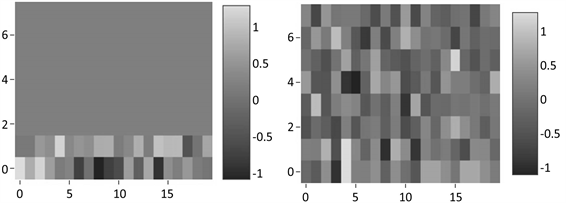(a) NTM内存示例 (b) L-NTM内存示例

Figure 2. Example of memory3.4. 改进的读取寻址机制

${w}_{t}^{c}\left(i\right)←\frac{\mathrm{exp}\left({\beta }_{t}\text{K}\left[{c}_{t},{S}_{t}\left(i\right)\right]\right)}{{\sum }_{j}\mathrm{exp}\left({\beta }_{t}\text{K}\left[{c}_{t},{S}_{t}\left(j\right)\right]\right)}$ #(9)

${w}_{t}\left(i\right)←{g}_{t}\left(i\right){w}_{t}^{c}+\left(1-{g}_{t}\left(i\right)\right){w}_{t}^{l}\left(i\right)$ #(10)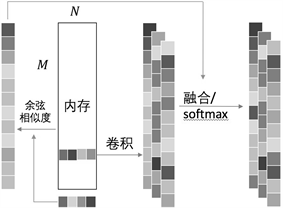4. 实验

bAbi数据集  是包含一系列推理任务的问答数据集，数据形式为故事陈述、问题和答案组成。我们使用了其中“Single Supporting Fact”和“Two Supporting Facts”两个子数据集用于NTM和L-NTM的比较，在“Single Supporting Fact”任务中，答案仅与陈述中的一句话有关联，只需一个事实支撑即可得到正确答案，在“Two Supporting Facts”中，为获取答案需要两句陈述支持，并且需要一定的推理才能完成。形式如图5所示。Figure 5. Examples of datasets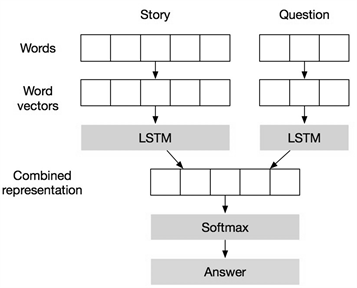(a) 序列到序列的模型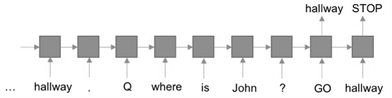(b) 陈述与问题分开式的模型

Figure 6. Structure of question answering models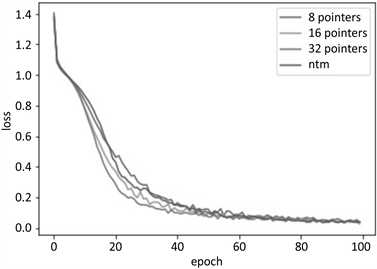Figure 7. Training results of “Single Supporting Fact”Table 1. Experiment results of LSTM, NTM and L-NTM

LSTM，这表明了具体的记忆存储可以提高推理任务的准确率。在简单的问答任务中，L-NTM将陈述的事实内容存储在内存空间中，在问题中序列中通过搜索存储内容进行推理，得到了更好的结果。因此在一些类似的序列任务中，L-NTM在一定程度上能够替代LSTM。

5. 结论

  Zeiler, M.D. and Fergus, R. (2014) Visualizing and Understanding Convolutional Networks. European Conference on Computer Vision, September 6 2014, 818-833. https://doi.org/10.1007/978-3-319-10590-1_53  Krizhevsky, A., Sutskever, I. and Hinton, G.E. (2012) Imagenet Classification with Deep Convolutional Neural Networks. Advances in Neural Information Processing Systems, 1097-1105.  Goodfellow, I., Bengio, Y. and Courville, A. (2016) Deep Learning. The MIT Press, Cambridge, 418-422.  Hinton, G.E. (1990) Mapping Part-Whole Hierarchies into Connectionist Networks. Artificial Intelligence, 46, 47-75. https://doi.org/10.1016/0004-3702(90)90004-J  Hochreiter, S. and Schmidhuber, J. (1997) Long Short-Term Memory. Neural Computation, 9, 1735-1780. https://doi.org/10.1162/neco.1997.9.8.1735  Santoro, A., Bartunov, S., Botvinick, M., Wierstra, D. and Lillicrap, T. (2016) One-Shot Learning with Memory- Augmented Neural Networks. arXiv preprint arXiv: 1605.06065  Weston, J., Chopra, S. and Bordes, A. (2014) Memory Networks. arXiv preprint arXiv:1410.3916  Sukhbaatar, S., Weston, J. and Fergus, R. (2015) End-to-End Memory Networks. Advances in Neural Information Pro- cessing Systems, 2440-2448.  Graves, A., Wayne, G. and Danihelka, I. (2014) Neural Turing Machines. arXiv preprint arXiv:1410.5401  Siegelmann, H.T. and Sontag, E.D. (1995) On the Computational Power of Neural Nets. Journal of Computer and System Sciences, 50, 132-150. https://doi.org/10.1006/jcss.1995.1013  Kurach, K., Andrychowicz, M. and Sutskever, I. (2015) Neural Random-Access Machines. arXiv preprint arXiv:1511.06392  Graves, A., Wayne, G., Reynolds, M., Harley, T., Danihelka, I., Grabska-Barwińska, A., Colmenarejo, S.G., Grefenstette, E., Ramalho, T., Agapiou, J. and Badia, A.P. (2016) Hybrid Computing Using a Neural Network with Dynamic External Memory. Nature, 538, 471-476. https://doi.org/10.1038/nature20101  Bahdanau, D., Cho, K. and Bengio, Y. (2014) Neural Machine Translation by Jointly Learning to Align and Translate. arXiv preprint arXiv:1409.0473  Mnih, V., Heess, N. and Graves, A. (2014) Recurrent Models of Visual Attention. Advances in Neural Information Processing Systems, 2204-2212.  LeCun, Y. and Bengio, Y. (1995) Convolutional Networks for Images, Speech, and Time Series. The Handbook of Brain Theory and Neural Networks, 3361, 1995.  Li, Y. (2017) Deep Reinforcement Learning: An Overview. arXiv preprint arXiv:1701.07274  Mnih, V., Kavukcuoglu, K., Silver, D., Rusu, A.A., Veness, J., Bellemare, M.G., Graves, A., Riedmiller, M., Fidjeland, A.K., Ostrovski, G. and Petersen, S. (2015) Human-Level Control through Deep Reinforcement Learning. Nature, 518, 529-533. https://doi.org/10.1038/nature14236  Reed, S. and De Freitas, N. (2015) Neural Programmer-Interpreters. arXiv preprint arXiv:1511.06279  Weston, J., Bordes, A., Chopra, S., Rush, A.M., van Merriënboer, B., Joulin, A. and Mikolov, T. (2015) Towards Ai- Complete Question Answering: A Set of Prerequisite Toy Tasks. arXiv preprint arXiv:1502.05698  Stroh, E. and Mathur, P. (2016) Question Answering Using Deep Learning.